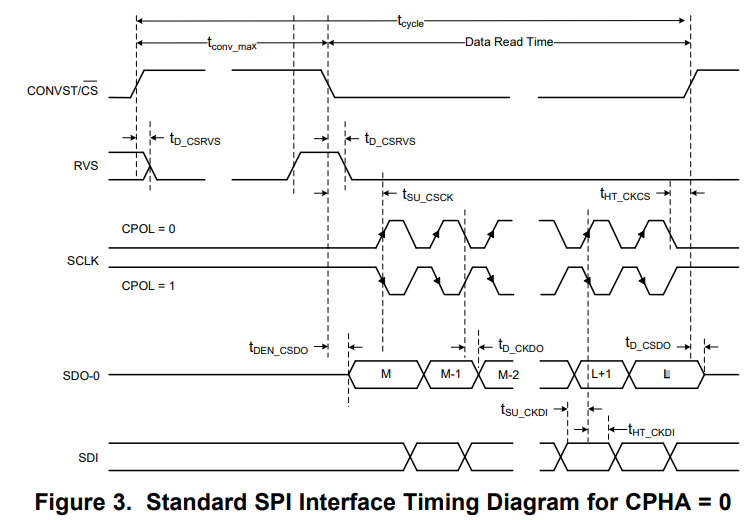If you have a related question, please click the "Ask a related question" button in the top right corner. The newly created question will be automatically linked to this question.

Hi team,

1. The datasheet mentions like below at 7.5.3 Output Data Word. Does this mean that, even if NOP command is issued, frame(F + 1) contains ADC's conversion result?

"For all other combinations, the output data word for frame (F+1) contains the latest 14-bit conversion result."

2. ADC's conversion result is MSB first or LSB first?

D[31:18] is Conversion result, but I am not sure conversion result is [13:0](MSB first) or [0:13](LSB first).

Regards,

Noriyuki Takahashi

• Noriyuki-san,

1. Correct.  See the timing for CPHA=0 as an example below, the procedure is

• The rising edge of CONVST/CS signal will initiate a data conversion.
• When the NOP command is issued and the CONVST/CS signal is forced to low, your controller will send out multiple clocks to the ADC. The ADC will shift out the the data converted in previous step to SDO. Your controller can read them in the same frame.
•  The CONVST/CS signal is pulled up to high when the data transfer is done. The rising edge of CONVST/CS signal will initiate another data conversion.

2.  MSB first, see the M on the SDO in the timing below also in the Figure 4/73/74 of DS.Regards,

Dale

• Dale,

Thanks!

In table 6 on the datasheet, D[5:4] is for Parity bits. Is it OK that D is for ADC output and D is for the entire output data frame?

Regards,

Noriyuki Takahashi

• Dale,

One more question.

In the description of PAR_EN, we can see below explanation. Does "the entire output data frame" mean D[31:0] including parity bits D[5:4] and D[3:0] or only D[31:6] in case of Table 6 in the datasheet? Then, how about in case of Table 7?

"The output data frame parity bit reflects an even parity signature for the entire output data frame, including the ADC output bits and any internal flags or register settings"

Regards,

Noriyuki Takahashi

• Noriyuki,

Q1: No. When the output data word only has ADC output data, the parity bits reflect an even parity for the ADC output bits only.

When the output data word includes the ADC output data and other internal flags or register settings, the parity bits reflect an even parity for the entire output data frame.

Q2: It includes entire bits D[31:0].

Regards,

Dale

• Hi Dale,

I am still not sure how to calculate the parity bits.

Could you review it? Could you also tell the definition of parity bits for ADC outputs and output data frame?

examples for parity bits.pptx

Regards,

Noriyuki Takahashi

• Hi Noriyuki,

I will get it back to you soon.

Best regards,

Dale

• Hi Noriyuki,

To be clear:

• First ADC parity bit:   this bit will only consider the ADC output bits for parity calculation.
• Second frame parity bit:   this bit will consider all bits in the output frame for parity calculation including ADC output bits and any internal flags or register settings also the ADC parity bit as well.

1.  ADC output parity - In this example, ADC output parity should be 1, right?

The output data frame parity - How should this parity bit be calculated? Parity bits[5:4] should be included in this calculation? Bits[3:0] is also included?

Answer:  See the clarification for the bit calculation above. This second frame parity bit is 0 based on the clarification. Bit[3:0] are all 0s, these bits do not affect the parity calculation.

2. ADC output parity - In this example, ADC output parity should be 0, right?

The output data frame parity - How should this parity bit be calculated? Parity bits[5:4] should be included in this calculation? Bits[3:0] is also included?

Answer:  See the clarification for the bit calculation above. This second frame parity bit is 0. Bit[3:0] are all 0s, these bits do not affect the parity calculation.

Regards,

Dale

• Dale,

Please let me make sure one more thing just in case.

Second frame parity bit, in case of the example I attached in the previous post,  D doesn't include D for parity bit calculation, right?

I mean, D[31:5] & D[3:0] are included in the calculation, right?

Regards,

Noriyuki Takahashi

• Hi Noriyuki,

The parity bits are calculated for even parity, and  the parity bits self are used for parity check to meet even parity.  Also, Bit[3:0] are all 0s, these bits do not affect the parity calculation.

Regards,

Dale